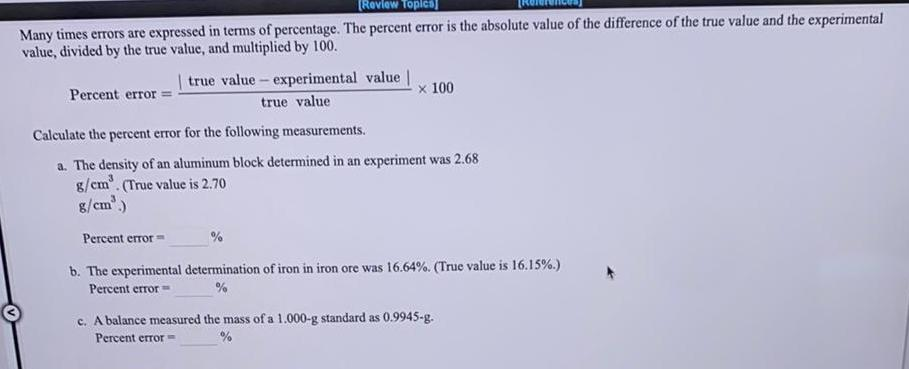Question:

# Many times errors are expressed in terms of percentage. The

Last updated: 8/10/2022Many times errors are expressed in terms of percentage. The percent error is the absolute value of the difference of the true value and the experimental value, divided by the true value, and multiplied by 100. Percent error=|true value-experimental value |/true value x 100 Calculate the percent error for the following measurements. a. The density of an aluminum block determined in an experiment was 2.68 g/cm³. (True value is 2.70 g/cm³.) b. The experimental determination of iron in iron ore was 16.64%. (True value is 16.15%.) Percent error= c. A balance measured the mass of a 1.000-g standard as 0.9945-g. Percent error=